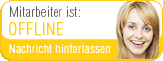## Course: Children demand basic mathematical knowledge

#### Scientific fundamentals of mathematics

none

##### Orientation

introductory and orientation period (STEOP)

##### Aim

The aim of this course is to convey the subject-related scientific fundamentals that form the basis for primary school didactics in mathematics. To this end, students develop and consolidate their basic knowledge as well as their mathematical abilities and skills with a focus on relevant mathematical content of primary school and secondary level 1.

##### Content
• Expansion of the individual action repertoire in the mathematical action dimensions (representation, interpretation; operation, calculation, construction; description, assumption, justification; modelling, problem solving)
• Inner-mathematical fundamentals in relevant sub-areas of mathematics
• Concrete, application-related use of technical terms and mathematical terminology

show details
back to overview